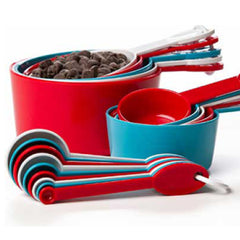Inspiring Cooks Since 1975.
Established in 1975.

# Cook's Resources — Measurement Guide1 Cup = 8 Fl. Ounces = 1/2 Pint = 237 ml. = 16 Tbsp. = 48 Tsp. 2 Cups = 16 Fl. Ounces = 1 Pint = 473 ml. 4 Cups = 32 Fl. Ounces = 1 Quart = 946 ml. 2 Pints = 32 Fl. Ounces = 1 Quart = .946 Liters 4 Quarts = 128 Fl. Ounces = 1 Gallon = 3.785 Liters

 1 Tsp. = 1/16 Fl. Ounce = 5 grams = about 5 ml. 1 Tbsp. = 1/2 Fl. Ounce = 15 grams = 15 ml. = 3 Tsp. = 1/16 Cup 2 Tbsp. = 1 Fl. Ounce = 30 grams = 29.6 ml. = 1/8 Cup 8 Tbsp. = 4 Fl. Ounces = 1/4 Pint = 118.5 ml. = 1/2 Cup

• To convert ounces to grams, multiply ounces by 28.35.
• To convert pounds to grams, multiply pounds by 453.59.

 3 Tsp. = 1 Tbsp. = 1/2 Ounce = 14.3 grams 2 Tbsp. = 1/8 Cup = 1 Ounce = 28.35 grams 4 Tbsp. = 1/4 Cup = 2 Ounces = 56.7 grams 5 1/3 Tbsp. = 1/3 Cup = 2.6 Ounces = 75.6 grams 8 Tbsp. = 1/2 Cup = 4 Ounces = 113.4 grams = 1 stick of butter 12 Tbsp. = 3/4 Cup = 6 Ounces = 170 grams = .375 lb. 32 Tbsp. = 2 Cups = 16 Ounces = 453.6 grams = 1 lb. 64 Tbsp. = 4 Cups = 32 Ounces = 907 grams = 2 lbs.
 1/5 Tsp. = 1 ml. 1 Tsp. = 5 ml. 1 Tbsp. = 15 ml. 1 Fl. Ounce = 30 ml. 1/5 Cup = 47 ml. 1 Cup = 237 ml. 2 Cups (1 pint) = 473 ml. 4 Cups (1 quart) = .95 Liter 4 Quarts (1 gal.) = 3.8 Liter
 1 Ounce = 28 grams 1 lb. = 454 grams
 1 ml. = 1/5 Tsp. 5 ml. = 1 Tsp. 15 ml. = 1 Tbsp. 100 ml. = 3.4 Fl. Ounces 240 ml. = 1 Cup 1 Liter = 34 Fl. Ounces = 4.2 Cups = 2.1 Pints = 1.06 Quarts = .26 Gal.
 1 gram = .035 Ounces 100 grams = 3.5 Ounces 500 grams = 1.10 lbs. 1 kg. = 2.205 lbs. = 35 Ounces

Free Shipping...

on orders of \$59 or more!

Visit Us...

at any of our PA stores

## Compare products

{"one"=>"Select 2 or 3 items to compare", "other"=>"{{ count }} of 3 items selected"}

Select first item to compare

Select second item to compare

Select third item to compare